# Multi-Step Equations with Variables on One Side and Both Sides Lesson Plan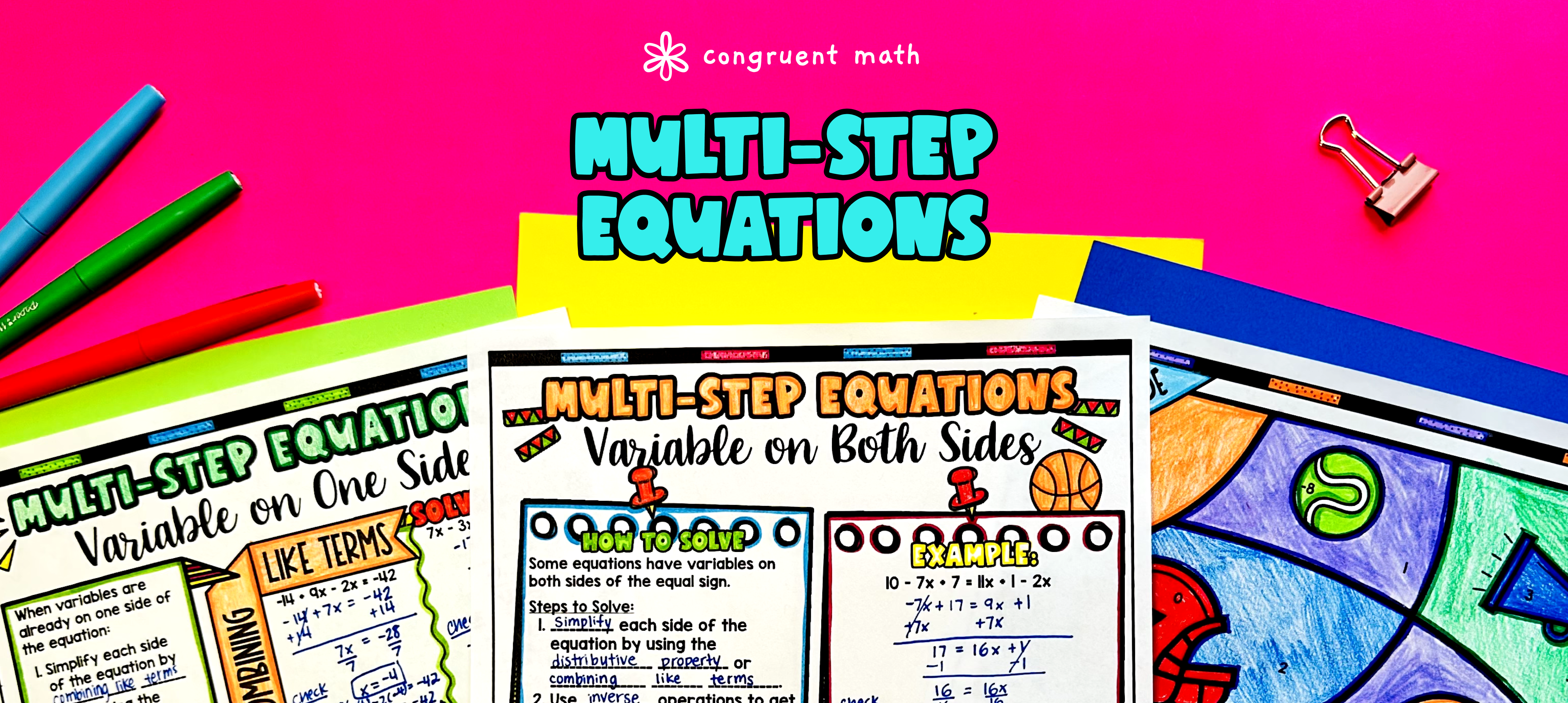Pin This

## Overview

Ever wondered how to teach multi-step equations with variables on one side and both sides in an engaging way to your eighth-grade students?

In this lesson plan, students will learn about solving multi-step equations with variables on one side and both sides and their real-life applications. They will solve these equations by combining like terms and using the distributive property. Through artistic and interactive guided notes, checks for understanding, a doodle, color by number activity, and a maze worksheet, students will gain a comprehensive understanding of multi-step equations.

The lesson culminates with a real-life example that explores how multi-step equations can be used in practical situations. Students will read and write about real-life uses of multi-step equations, connecting the math concepts to the real world.

With this lesson plan, students will not only develop their problem-solving skills but also engage with the content in a creative and enjoyable way. They will be able to apply their knowledge of multi-step equations to real-life situations, making the math concepts more relevant and meaningful.

## Get the Lesson Materials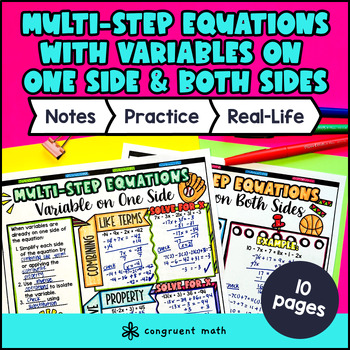\$4.25

## Learning Objectives

After this lesson, students will be able to:

• Solve multi-step equations with variables on one side.

• Solve multi-step equations with variables on both sides.

• Simplify parts of equations by combining like terms and using the distributive property

• Apply the skills learned in solving multi-step equations to real-life math problems.

## Prerequisites

Before this lesson, students should be familiar with:

• Solving one-step equations

• Understanding of basic operations (addition, subtraction, multiplication, and division)

• Knowledge of what like terms are

## Key Vocabulary

• Multi-step equations

• Combining like terms

• Distributive property

• Inverse operations

• Coefficients

## Procedure

### Introduction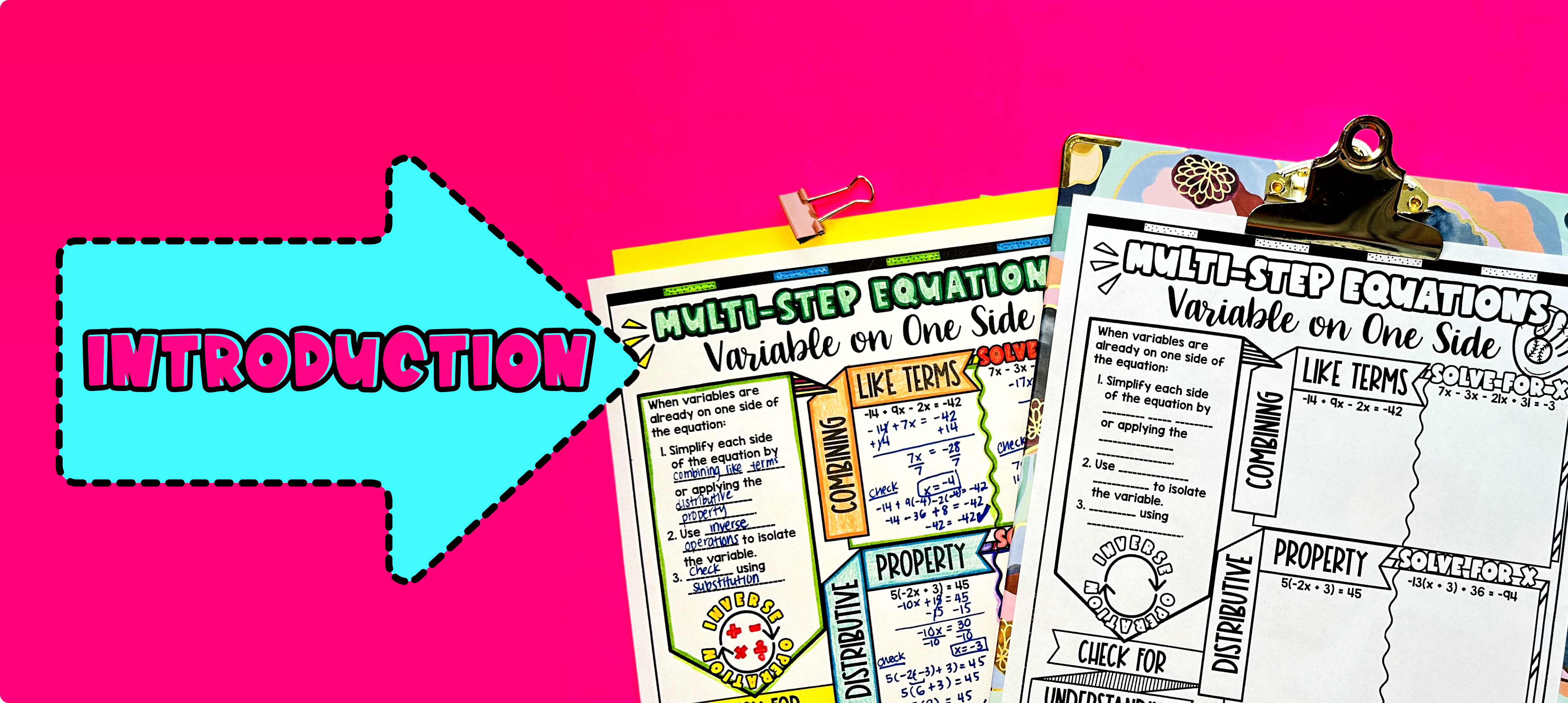Pin This

As a hook, ask students why solving multi-step equations is important in real life. You can give examples such as budgeting, calculating distances, or determining the number of items needed for a recipe. Refer to the last page of the guided notes for ideas.

Use the first page of the guided notes to introduce solving multi-step equations with variables on one side. Walk through the key points of the topic, including combining like terms, applying the distributive property, and using inverse operations to isolate the variable. Then, move onto the next page of the guided notes to discuss how to solve multi0step equations with variables on both sides.

Based on student responses, reteach concepts that students need extra help with. If your class has a wide range of proficiency levels, you can pull out students for reteaching, and have more advanced students begin work on the practice exercises.

### Practice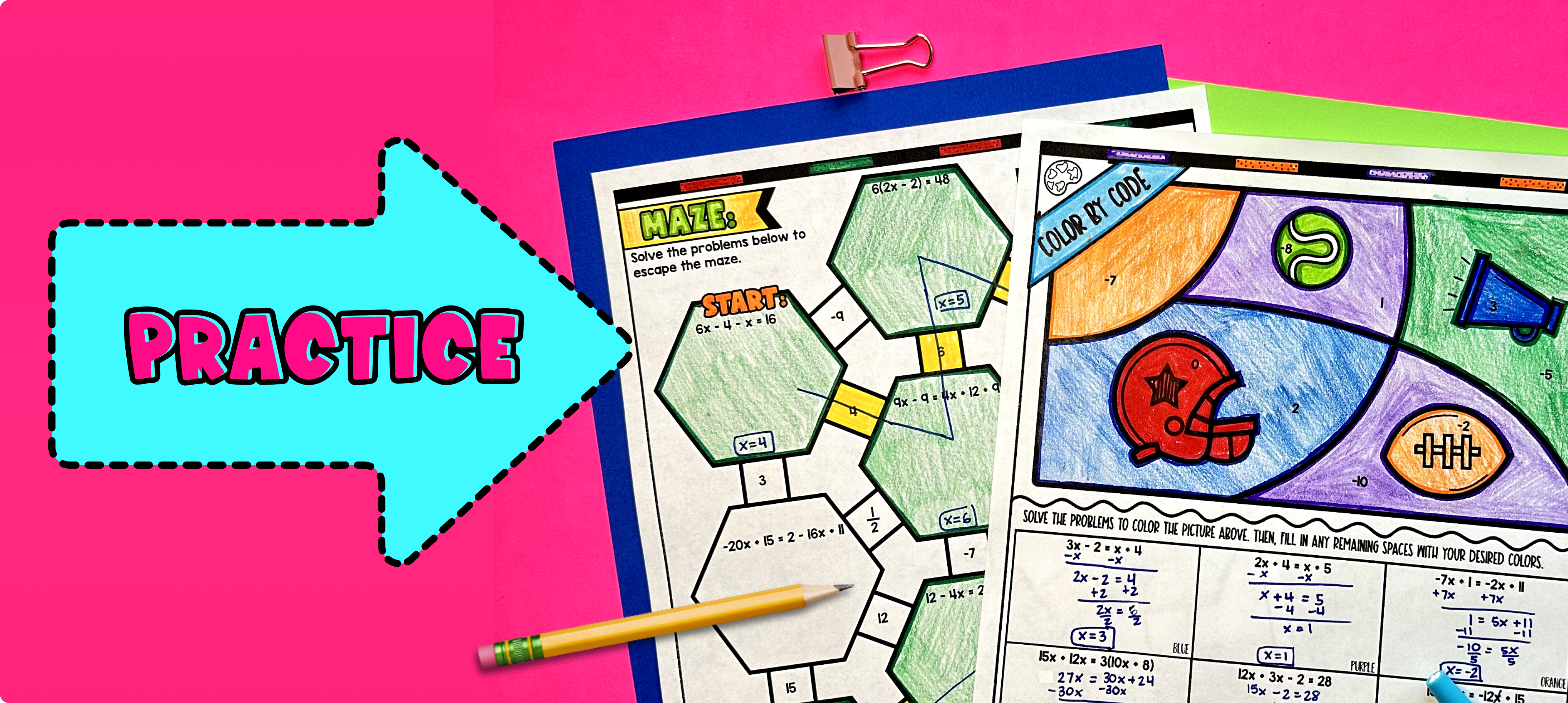Pin This

Have students practice solving multi step equations with variables on one side and both sides using the practice worksheet included in the resource. This guided notes packet includes a color by code activity and a maze activity.

Walk around the classroom to answer any questions that students may have and provide support as needed.

For fast finishers, they can further practice their skills by completing the real-life application activity included in the resource. This activity requires students to read and write about real-life situations that involve solving multi-step equations. You can assign it as homework for the remainder of the class, allowing students to apply their knowledge in a practical context.

### Real-Life Application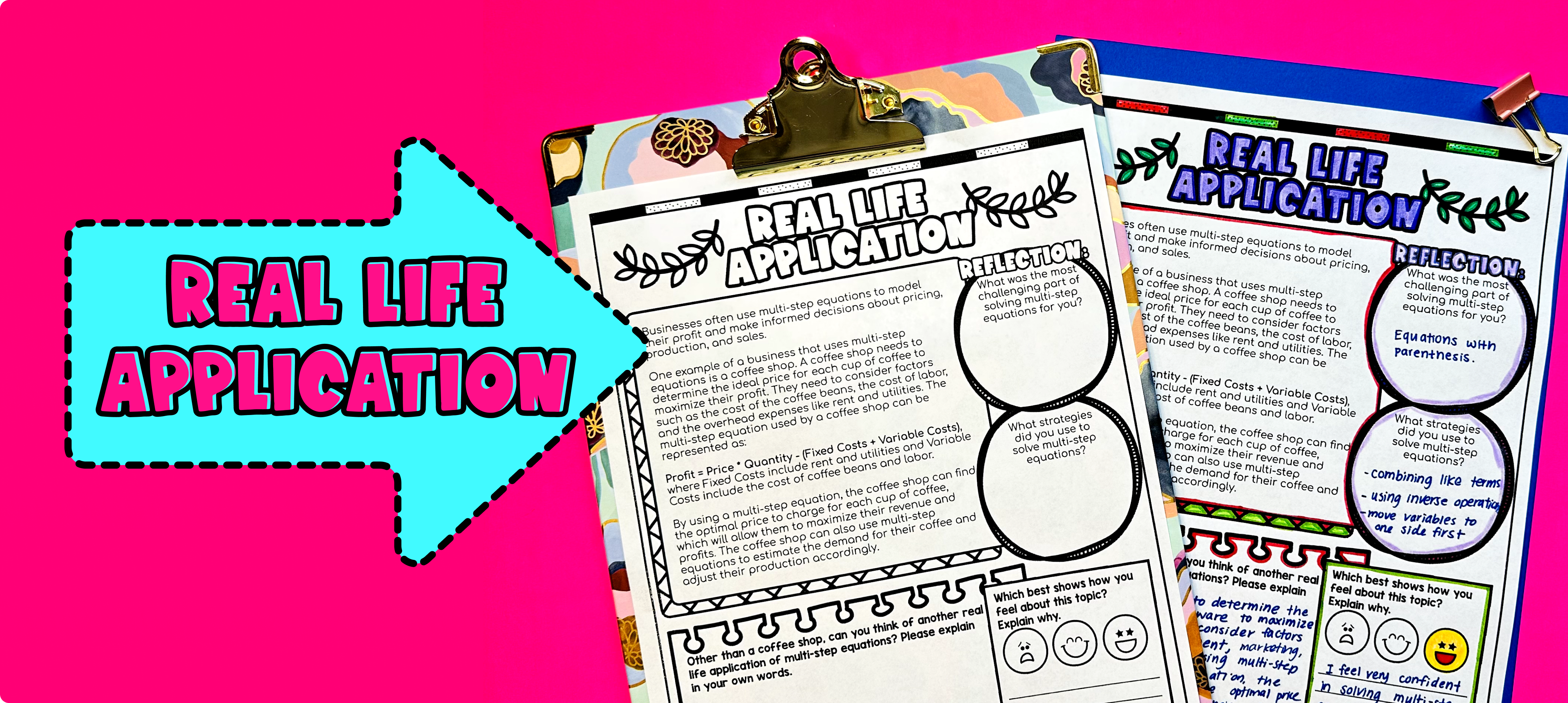Pin This

Bring the class back together, and introduce the concept of real-world applications of multi-step equations. Discuss how solving multi-step equations can be used in various scenarios to solve real-life problems. For example, solving multi-step equations can help determine the amount of time it takes to complete a task, the cost of an item, or the distance traveled.

Use the last page of the guided notes resource titled "real life application" to explore a detailed example of real-world applications of multi-step equations. Encourage students to think critically and creatively about how they can apply their math skills to solve practical problems they may encounter in the future. Students can also fill out the reflection section on the last page of the guided notes resource to assess their learning.

## Extensions

If you’re looking for digital practice for comparing and ordering integers and absolute values, try my Pixel Art activities in Google Sheets. Every answer is automatically checked, and correct answers unlock parts of a mystery picture. It’s incredibly fun, and a powerful tool for differentiation.

Here are some activities to explore: